Anzeige

# .

25. Nov 2008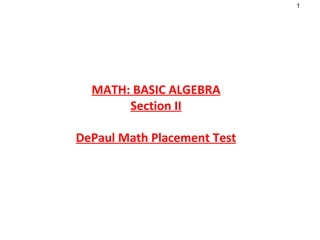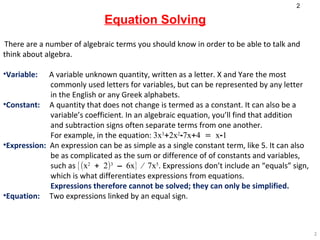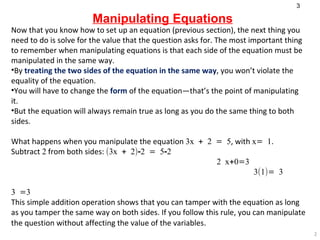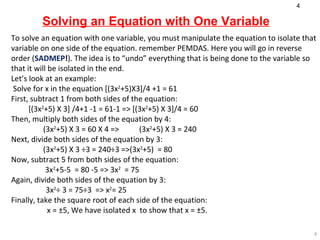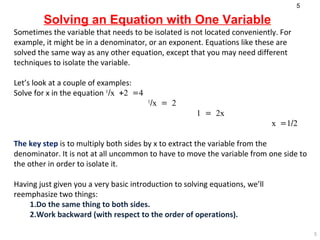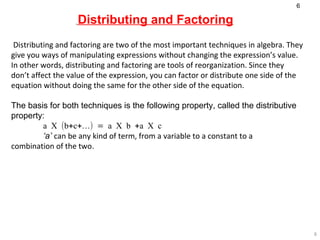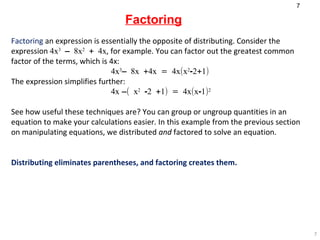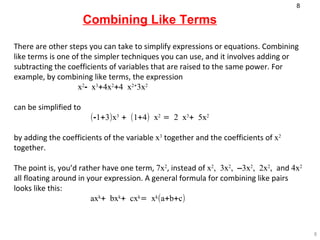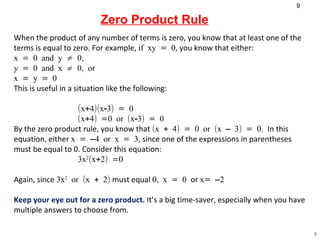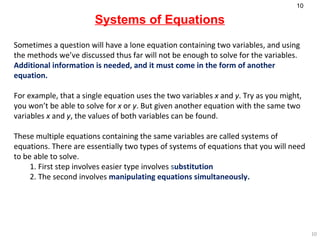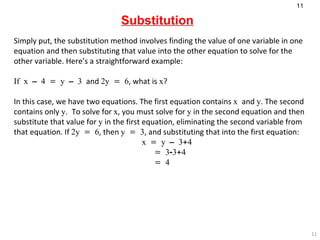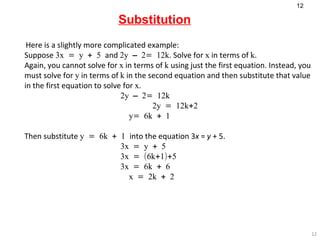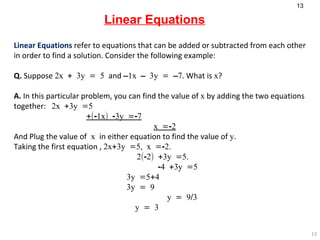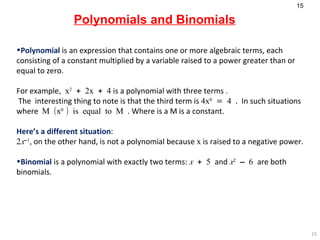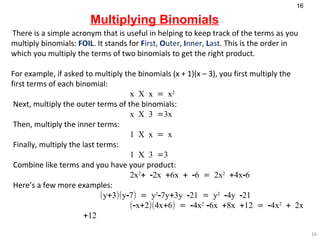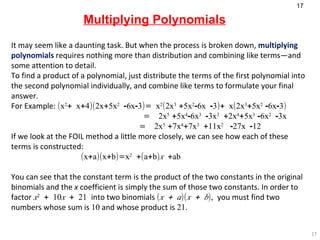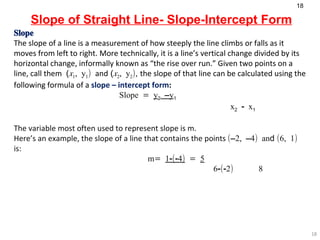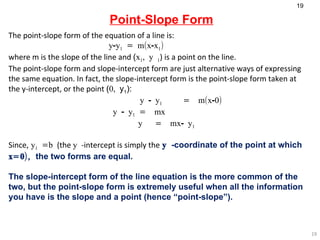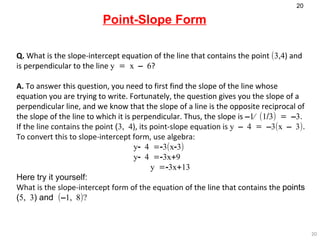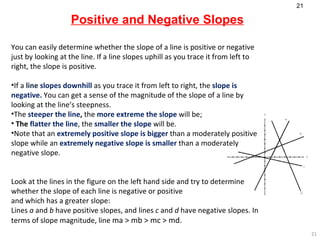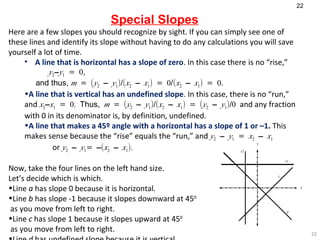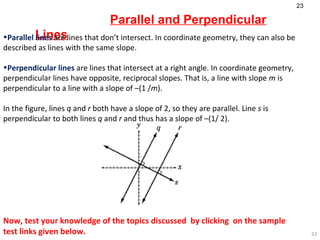1 von 23
Anzeige

### .

1. MATH: BASIC ALGEBRA Section II DePaul Math Placement Test
2. Solving an Equation with One Variable To solve an equation with one variable, you must manipulate the equation to isolate that variable on one side of the equation. remember PEMDAS. Here you will go in reverse order ( SADMEP ! ). The idea is to “undo” everything that is being done to the variable so that it will be isolated in the end. Let’s look at an example: Solve for x in the equation [(3x 2 +5)X3]/4 +1 = 61 First, subtract 1 from both sides of the equation: [(3x 2 +5) X 3] /4+1 -1 = 61-1 => [(3x 2 +5) X 3]/4 = 60 Then, multiply both sides of the equation by 4: (3x 2 +5) X 3 = 60 X 4 => (3x 2 +5) X 3 = 240 Next, divide both sides of the equation by 3: (3x 2 +5) X 3 ÷3 = 240÷3 =>(3x 2 +5) = 80 Now, subtract 5 from both sides of the equation: 3x 2 +5-5 = 80 -5 => 3x 2 = 75 Again, divide both sides of the equation by 3 : 3x 2 ÷ 3 = 75÷3 => x 2 = 25  Finally, take the square root of each side of the equation: x = ±5 , We have isolated x to show that x = ±5 .
3. Distributing and Factoring Distributing and factoring are two of the most important techniques in algebra. They give you ways of manipulating expressions without changing the expression’s value. In other words, distributing and factoring are tools of reorganization. Since they don’t affect the value of the expression, you can factor or distribute one side of the equation without doing the same for the other side of the equation. The basis for both techniques is the following property, called the distributive property: a X (b+c+…) = a X b +a X c   ‘ a’ can be any kind of term, from a variable to a constant to a combination of the two .
4. Factoring Factoring an expression is essentially the opposite of distributing. Consider the expression 4x 3 – 8x 2 + 4x , for example. You can factor out the greatest common factor of the terms, which is 4x : 4x 3 – 8x +4x = 4x(x 2 -2+1) The expression simplifies further: 4x –( x 2 -2 +1) = 4x(x-1) 2   See how useful these techniques are? You can group or ungroup quantities in an equation to make your calculations easier. In this example from the previous section on manipulating equations, we distributed and factored to solve an equation. Distributing eliminates parentheses, and factoring creates them.
5. Combining Like Terms There are other steps you can take to simplify expressions or equations. Combining like terms is one of the simpler techniques you can use, and it involves adding or subtracting the coefficients of variables that are raised to the same power. For example, by combining like terms, the expression x 2 - x 3 +4x 2 +4 x 2+ 3x 2   can be simplified to (-1+3)x 3 + (1+4) x 2 = 2 x 3 + 5x 2   by adding the coefficients of the variable x 3 together and the coefficients of x 2 together. The point is, you’d rather have one term, 7x 2 , instead of x 2 , 3x 2 , –3x 2 , 2x 2 , and 4x 2 all floating around in your expression. A general formula for combining like pairs looks like this: ax k + bx k + cx k = x k (a+b+c)
6. Zero Product Rule   When the product of any number of terms is zero, you know that at least one of the terms is equal to zero. For example, if xy = 0 , you know that either: x = 0 and y ≠ 0, y = 0 and x ≠ 0, or x = y = 0 This is useful in a situation like the following: (x+4)(x-3) = 0 (x+4) =0 or (x-3) = 0 By the zero product rule, you know that (x + 4) = 0 or (x – 3) = 0. In this equation, either x = –4 or x = 3 , since one of the expressions in parentheses must be equal to 0. Consider this equation: 3x 2 (x+2) =0   Again, since 3x 2 or (x + 2) must equal 0, x = 0 or x= –2 Keep your eye out for a zero product. I t’s a big time-saver, especially when you have multiple answers to choose from.
7. Substitution Simply put, the substitution method involves finding the value of one variable in one equation and then substituting that value into the other equation to solve for the other variable. Here’s a straightforward example: If x – 4 = y – 3 and 2y = 6 , what is x ? In this case, we have two equations. The first equation contains x and y . The second contains only y. To solve for x , you must solve for y in the second equation and then substitute that value for y in the first equation, eliminating the second variable from that equation. If 2y = 6 , then y = 3 , and substituting that into the first equation: x = y – 3+4 = 3-3+4 = 4
8. Substitution Here is a slightly more complicated example: Suppose 3x = y + 5 and 2y – 2= 12k . Solve for x in terms of k . Again, you cannot solve for x in terms of k using just the first equation. Instead, you must solve for y in terms of k in the second equation and then substitute that value in the first equation to solve for x .   2y – 2= 12k 2y = 12k+2 y= 6k + 1   Then substitute y = 6k + 1 into the equation 3 x = y + 5. 3x = y + 5 3x = (6k+1)+5 3x = 6k + 6 x = 2k + 2
9. Linear Equations Linear Equations refer to equations that can be added or subtracted from each other in order to find a solution. Consider the following example: Q. Suppose 2x + 3y = 5 and –1x – 3y = –7 . What is x ? A. In this particular problem, you can find the value of x by adding the two equations together: 2x +3y =5 +(-1x) -3y =-7 x =-2 And Plug the value of x in either equation to find the value of y . Taking the first equation , 2x+3y =5, x =-2. 2(-2) +3y =5. -4 +3y =5 3y =5+4 3y = 9 y = 9/3 y = 3
10. Linear Equations Here’s another example: Q. 2x + 3y = –6 and –4x + 16y = 13 . What is the value of y ? A. The question asks you to solve for y , which means that you should find a way to eliminate one of the variables by adding or subtracting the two equations. 4x is simply twice 2x , so by multiplying the first equation by 2, you can then add the equations together to find y. 2 X (2x + 3y = –6) = 4x + 6y = –12   Now add the equations and solve for y. 4x +6y =-12 +(-4x) +16y = 13 22y = 1 y= 1/22
11. Multiplying Polynomials It may seem like a daunting task. But when the process is broken down, multiplying polynomials requires nothing more than distribution and combining like terms—and some attention to detail. To find a product of a polynomial, just distribute the terms of the first polynomial into the second polynomial individually, and combine like terms to formulate your final answer. For Example: (x 2 + x+4)(2x+5x 2 -6x-3)= x 2 (2x 3 +5x 2 -6x -3)+ x(2x 3 +5x 2 -6x-3) = 2x 5 +5x 4 -6x 3 -3x 3 +2x 4 +5x 3 -6x 2 -3x = 2x 5 +7x 4 +7x 3 +11x 2 -27x -12 If we look at the FOIL method a little more closely, we can see how each of these terms is constructed: (x+a)(x+b)=x 2 +(a+b) x +ab   You can see that the constant term is the product of the two constants in the original binomials and the x coefficient is simply the sum of those two constants. In order to factor x 2 + 10 x + 21 into two binomials ( x + a )( x + b ), you must find two numbers whose sum is 10 and whose product is 21 .
12. Slope of Straight Line- Slope-Intercept Form Slope The slope of a line is a measurement of how steeply the line climbs or falls as it moves from left to right. More technically, it is a line’s vertical change divided by its horizontal change, informally known as “the rise over run.” Given two points on a line, call them ( x 1 , y 1 ) and ( x 2 , y 2 ) , the slope of that line can be calculated using the following formula of a slope – intercept form : Slope = y 2 –y 1 x 2 - x 1 The variable most often used to represent slope is m. Here’s an example, the slope of a line that contains the points (–2, –4) an d (6, 1) is: m= 1-(-4) = 5 6-(-2) 8
13. Point-Slope Form The point-slope form of the equation of a line is: y-y 1 = m(x-x 1 ) where m is the slope of the line and ( x 1 , y 1 ) is a point on the line. The point-slope form and slope-intercept form are just alternative ways of expressing the same equation. In fact, the slope-intercept form is the point-slope form taken at the y-intercept, or the point ( 0, y 1 ): y - y 1 = m(x-0) y - y 1 = mx y = mx- y 1 Since, y 1 =b (the y -intercept is simply the y -coordinate of the point at which x=0), the two forms are equal. The slope-intercept form of the line equation is the more common of the two, but the point-slope form is extremely useful when all the information you have is the slope and a point (hence “point-slope”).
14. Point-Slope Form Q. What is the slope-intercept equation of the line that contains the point (3,4 ) and is perpendicular to the line y = x – 6 ? A. To answer this question, you need to first find the slope of the line whose equation you are trying to write. Fortunately, the question gives you the slope of a perpendicular line, and we know that the slope of a line is the opposite reciprocal of the slope of the line to which it is perpendicular. Thus, the slope is –1⁄ (1/3) = –3 . If the line contains the point ( 3, 4 ), its point-slope equation is y – 4 = –3(x – 3) . To convert this to slope-intercept form, use algebra: y- 4 =-3(x-3) y- 4 =-3x+9 y =-3x+13 Here try it yourself: What is the slope-intercept form of the equation of the line that contains the points ( 5, 3 ) and (–1, 8)?
Anzeige